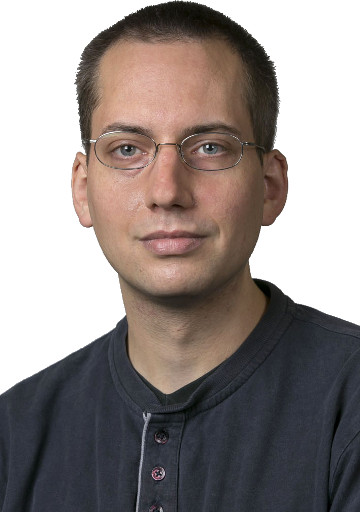# Tobias RossmannI am a Lecturer in Mathematics in the School of Mathematics, Statistics & Applied Mathematics at NUI Galway.

## Research interests

My research is in the field of algebra, mostly of the asymptotic or computational kind. In recent years, my main focus has been on zeta functions arising from algebraic counting problems such as the enumeration of subgroups, representations, or conjugacy classes.

## Papers

### Journal articles

1. Irreducibility testing of finite nilpotent linear groups. J. Algebra 324 (2010), 1114–1124. (preprint)
2. Primitivity testing of finite nilpotent linear groups LMS J. Comput. Math. 14 (2011), 87–98. (preprint)
3. Computing topological zeta functions of groups, algebras, and modules, I. Proc. Lond. Math. Soc. (3) 110 (2015), no. 5, 1099–1134. (preprint)
4. Computing topological zeta functions of groups, algebras, and modules, II. J. Algebra 444 (2015), 567–605. (preprint)
5. Topological representation zeta functions of unipotent groups. J. Algebra 448 (2016), 210–237. (preprint)
6. Primitive finite nilpotent linear groups over number fields. J. Algebra 451 (2016), 248–267. (preprint)
7. Enumerating submodules invariant under an endomorphism. Math. Ann. 368 (2017), no. 1, 391–417. (preprint, view online)
8. Computing local zeta functions of groups, algebras, and modules. Trans. Amer. Math. Soc. 370 (2018), no. 7, 4841–4879. (preprint)
9. The average size of the kernel of a matrix and orbits of linear groups. Proc. Lond. Math. Soc. (3) 117 (2018), no. 3, 574–616. (preprint)
10. Stability results for local zeta functions of groups, algebras, and modules, Math. Proc. Camb. Phil. Soc. 165 (2018), no. 3, 435–444. (preprint)
11. The average size of the kernel of a matrix and orbits of linear groups, II: duality, J. Pure Appl. Algebra 224 (2020), no. 4, 106203, 28 pages. (preprint)

### Book chapters

1. Periodicities for graphs of p-groups beyond coclass (with Bettina Eick). In: Kappe et al. (eds), Computational group theory and the theory of groups, II. Contemp. Math. 511 (2010), 11–23. (preprint)
2. A Framework for Computing Zeta Functions of Groups, Algebras, and Modules. In: Böckle et al. (eds), Algorithmic and Experimental Methods in Algebra, Geometry and Number Theory (2017), Springer-Verlag, 561–586. (preprint)

### Preprints

1. Groups, graphs, and hypergraphs: average sizes of kernels of generic matrices with support constraints (with Christopher Voll), 109 pages. (preprint)

## Software

1. fwtree — computing trees related to some pro-p-groups of finite width (with Bettina Eick) (2009).
2. finn — computing with finite nilpotent linear groups (2010–2011).
3. Zeta — computing zeta functions of groups, algebras, and modules (2014–present).

## Theses

1. p-Groups: Rank, Width, and Obliquity. Diploma thesis, TU Braunschweig (2007).
2. Algorithms for Nilpotent Linear Groups. PhD thesis, NUI Galway (2011). (PDF)
3. Zeta Functions of Groups, Algebras, and Modules. Habilitation thesis, Bielefeld University (2017). (Title page and introduction: PDF)

## Reports

1. Computing with nilpotent linear groups. Oberwolfach Reports 8 (2011), no. 3, Computational Group Theory, 2121. (preprint)
2. Computing zeta functions of groups, algebras, and modules. Oberwolfach Reports 13 (2016), no. 3, Computational Group Theory, 2144–2145. (preprint)

## Selected talks

 Since 2018 Lecturer in Mathematics, NUI Galway 2016–2018 Feodor Lynen Postdoctoral Fellow (Alexander von Humboldt-Foundation), University of Auckland. Host: Eamonn O'Brien 2011–2016 Postdoc, Bielefeld University. DFG-funded project Toroidal methods for computing zeta functions of groups and rings (2013–2016) within the Priority Programme “Algorithmic and Experimental Methods in Algebra, Geometry and Number Theory”.

## Education

 2017 Habilitation in Mathematics, Bielefeld University 2011 PhD in Mathematics, National University of Ireland, Galway 2008 Diplom in Mathematics, Technische Universität Braunschweig

## Conferences organised

1. (Upcoming:) Groups in Galway meets the Irish Geometry Conference (with John Burns, Angela Carnevale, and Martin Kerin), 14–16 May 2020.
2. Groups in Galway 2019 (with Graham Ellis), 10–11 May 2019.
3. Groups in Galway 2018 (with Dane Flannery and Kevin Jennings), 18–19 May 2018.

## Teaching 2019/2020

1. Computer Science (CS102/CS103) (Semester 1)
2. Linear Algebra (MA313) (Semester 1)
3. Cryptography (CS402/MA492) (Semester 2)

## Contact

Tobias Rossmann
School of Mathematics, Statistics and Applied Mathematics
National University of Ireland, Galway
Galway
H91 TK33
Ireland

Office: Áras de Brún, room ADB-1006
Phone: +353 91 492043
E-mail: tobias.rossmann (at) nuigalway.ie